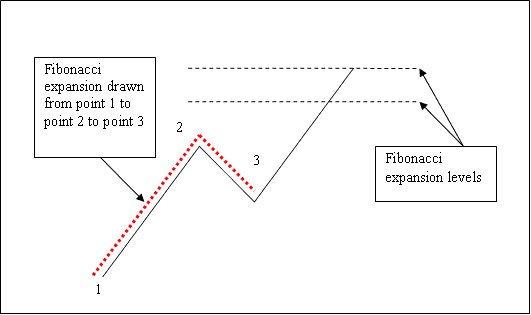# How to Analyze 61.8 Indices Trading Fibonacci Expansion Level

We useFibonacci expansionlevels to estimate where the indices trend movement will reach. There are 2 important Fibonacci expansion levels; 61.8% and 100% Indices Trading Fibonacci Expansion Levels, these are used for taking profit.

## 61.8 Indices Trading Fib Expansion Level

61.8 Indices Trading Fib Expansion Level is the most commonly used indices trading profit taking level when trading using the Indices Trading Fibonacci Expansion Indicator.

To draw Indices Trading Fibonacci Expansion levels on the stock indices chart we wait until the stock indexes price retracement is complete and the stock indexes price starts to move in the original direction of the Indices trend. Where the stock indexes price retracement reaches is used as chart point 3. Indices Trading Fibonacci Expansion Indicator is drawn using three chart points as shown on the stock indexes trading example explained and illustrated below of how to draw the Indices Trading Fibonacci Expansion Indicator Tool.

The Fibonacci expansion example explained and illustrated below shows the 3 Chart Points where the Fibonacci expansion indicator is drawn, marked as Chart point 1, Chart point 2 and Chart point 3.

Chart point 1 is where the indices trend started, Chart point 2 is where the indices trend pulled back and retraced and Chart point 3 is where the stock indices retracement reached as shown on the Indices Trading Fibonacci Expansion Indicator Tool example explained and illustrated below.

The First Indices Trading Fibonacci Expansion Level is the 61.8 Indices Trading Fib Expansion Level shown Below Used to Set Take Profit Indices Trading OrdersIndices Trading Fibonacci Expansion Level is the 61.8 Indices Trading Fib Expansion Level shown Below Used to Set Take Profit Indices Trading Orders

Please note where these Indices Trading Fibonacci Expansion levels are drawn - Indices Trading Fibonacci Expansion levels are drawn above the Fibonacci indicator, these are the chart points where a indices trader will set the take profit orders using these Indices Trading Fibonacci Expansion Levels - 61.8% Indices Trading Fibonacci Expansion Levels.